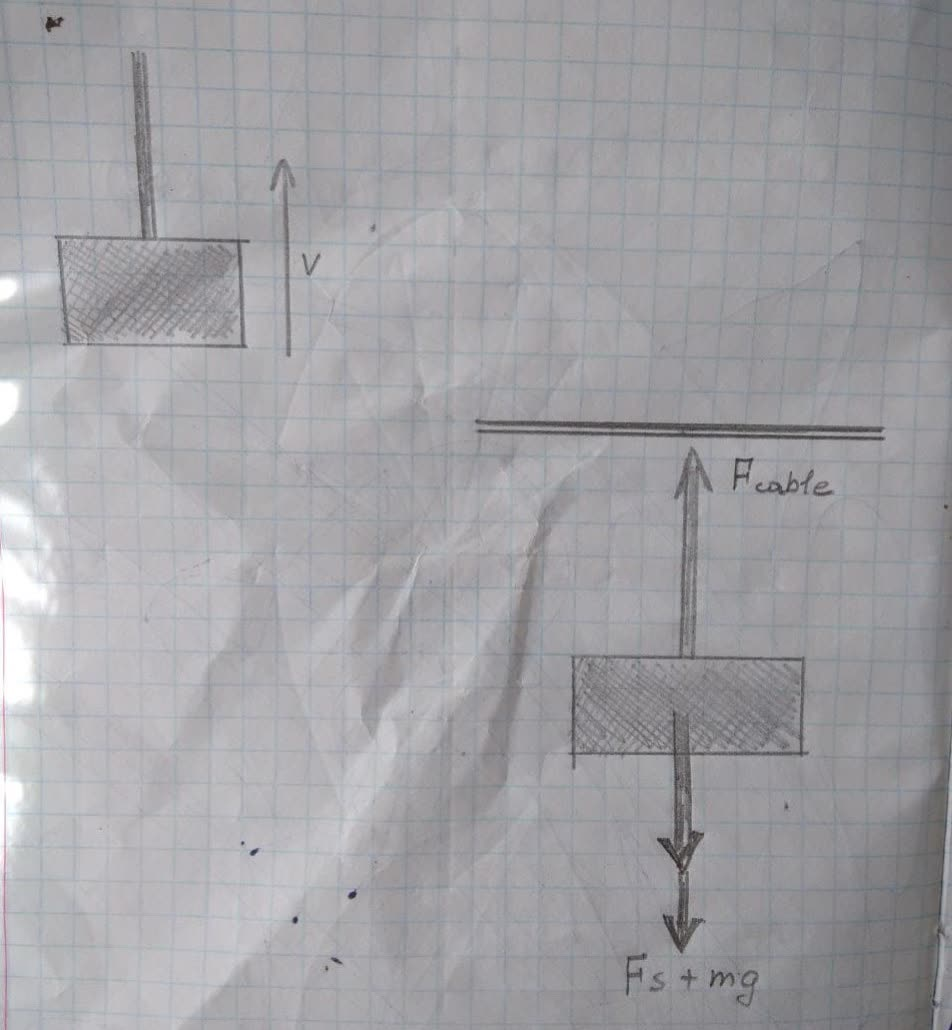Ernest Ryland

2022-01-10

a) Calculate the work done on a 1500-kg elevator car by its cable to lift it 40.0 m at constant speed, assuming friction averages 100 N.
b) What is the work done on the lift by the gravitational force in this process?
c) What is the total work done on the lift?GaceCoect5v

Expert

Given:
${m}_{car}=1500kg$
$\mathrm{△}y=40m$
${F}_{s}=100N$
(a)
First of all, we need to find the force of the cable.
Noting that the friction force always working in the opposite direction of the motion of the object.
We know, from Newton's second law, that
$\sum {F}_{y}={F}_{cable}-mg-{F}_{s}=m{a}_{y}$
And we know that ${a}_{y}=0$ since the velocity is constant.
hence,
${F}_{cable}-mg-{F}_{s}=0$
${F}_{cable}=mg+{F}_{s}$
We know that the work done by some force is given by
$W=Fd\mathrm{cos}\theta$
so the work done by the cable force on the car during this process is given by
${W}_{cable}={F}_{cable}\mathrm{△}y{\mathrm{cos}0}^{\circ }$
Plug from (1), and noting that cos ${0}^{\circ }=1.0$
${W}_{cable}=\left(mg+{F}_{s}\right)\mathrm{△}y$
Now plug the given;
${W}_{cable}=\left[\left(1500x9.8\right)+100\right]×40$
${W}_{cable}=5.92x{10}^{\circ }J$
(b)
The work done by the gravitational force is given by
${W}_{cable}={F}_{g}d\mathrm{cos}\theta$
${W}_{cable}=mg\mathrm{△}y\mathrm{cos}\theta$
Noting that \theta= 180° since the angle between the gravitational force and the velocity is ${180}^{\circ }$
Hence,
${W}_{gravity}=mg\mathrm{△}y{\mathrm{cos}180}^{\circ }$
Noting that cos ${180}^{\circ }=-1$
${W}_{gravity}=mg\mathrm{△}y$
Plug the given;
${W}_{gravity}=-1500×9.8×40$
${W}_{gravity}=-5.88×{10}^{5}J$
(c)
The total work done on the lift is the work done by the net force exerted on the car during this process.
Hence,
${W}_{tot}={F}_{net}\theta y$
We know that the net force exerted on the car during this process is zero since the car is moving at a constant speed (see part a above).
So,
${W}_{tot}=0×40$
${W}_{tot}=0J$Beverly Smith

Expert

The weight of the elevator is
$W=\left(1500kg\right)×\left(9.8\frac{m}{{s}^{2}}\right)=1.47×{10}^{4}N$
Because the frictional resistance is R = 100 N, the tension in the cable for dynamic equilibrium is
$T=W+R=1.47×{10}^{4}+100=1.48×{10}^{4}N$
By definition, the work done in lifting the elevator by 40 m is
$\left(1.48×{10}^{4}N\right)×\left(40m\right)=5.92×{10}^{5}J=592kJ$
Answer:$592kJ\left(\phantom{\rule{1em}{0ex}}\text{or}\phantom{\rule{1em}{0ex}}5.92×{0}^{5}J\right)$
W= 1500g
R=100Nstar233

Expert

Given
mass of elevator m=1500 kg
Lifting height =40 m
Friction Force F=100 N
The cable has to work against gravitational force and friction Force
Increase in Potential Energy of Elevator =mgh=1500$×$9.8$×$40=588 kJ
thus work done by gravitational force = 588 kJ
Work done against friction force = F * x =100 * 40=4 kJ
Total Work done by the cable = 592 kJ

Do you have a similar question?